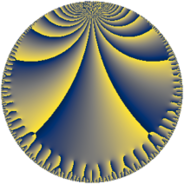# Properties

 Label 95.11.nLevel $95$ Weight $11$ Character orbit 95.n Rep. character $\chi_{95}(21,\cdot)$ Character field $\Q(\zeta_{18})$ Dimension $396$ Newform subspaces $1$ Sturm bound $110$ Trace bound $0$

# Related objects

## Defining parameters

 Level: $$N$$ $$=$$ $$95 = 5 \cdot 19$$ Weight: $$k$$ $$=$$ $$11$$ Character orbit: $$[\chi]$$ $$=$$ 95.n (of order $$18$$ and degree $$6$$) Character conductor: $$\operatorname{cond}(\chi)$$ $$=$$ $$19$$ Character field: $$\Q(\zeta_{18})$$ Newform subspaces: $$1$$ Sturm bound: $$110$$ Trace bound: $$0$$

## Dimensions

The following table gives the dimensions of various subspaces of $$M_{11}(95, [\chi])$$.

Total New Old
Modular forms 612 396 216
Cusp forms 588 396 192
Eisenstein series 24 0 24

## Trace form

 $$396 q + 132 q^{3} - 1830 q^{4} + 3066 q^{6} - 95436 q^{9} + O(q^{10})$$ $$396 q + 132 q^{3} - 1830 q^{4} + 3066 q^{6} - 95436 q^{9} - 93750 q^{10} + 2985984 q^{12} - 2110026 q^{13} + 1224960 q^{14} - 6809430 q^{16} + 532380 q^{17} - 7959948 q^{19} - 9580518 q^{21} - 31211136 q^{22} - 13532700 q^{23} + 21417984 q^{24} - 159720 q^{26} - 183408156 q^{27} + 144654336 q^{28} - 140983194 q^{29} - 119625000 q^{30} + 122776776 q^{31} + 393148500 q^{32} - 359004150 q^{33} - 578370492 q^{34} - 59812500 q^{35} - 640932912 q^{36} + 503327622 q^{38} + 1302975096 q^{39} - 96000000 q^{40} - 1194423528 q^{41} - 3390185778 q^{42} - 670104582 q^{43} + 2150443914 q^{44} + 3016431090 q^{46} - 151507770 q^{47} + 364629606 q^{48} - 8225668806 q^{49} + 3009006312 q^{51} - 2363609406 q^{52} - 4515798744 q^{53} - 9311985042 q^{54} + 952636938 q^{57} + 4039140864 q^{58} + 5041664862 q^{59} - 2779312500 q^{60} + 5128963260 q^{61} - 4033454760 q^{62} - 12114094326 q^{63} + 11990621196 q^{64} - 3916687500 q^{65} - 1806217476 q^{66} + 6379678362 q^{67} + 18479546670 q^{68} + 14567082498 q^{69} - 1621125000 q^{70} - 1788156144 q^{71} - 16923552852 q^{72} - 26786299530 q^{73} + 15012453948 q^{74} + 18346685868 q^{76} + 6003303804 q^{77} + 69668310660 q^{78} - 9538312266 q^{79} + 5565750000 q^{80} + 2012656020 q^{81} + 30701977302 q^{82} - 21873008760 q^{83} - 51655671540 q^{84} - 14398500000 q^{85} - 14081837694 q^{86} - 17926323216 q^{87} + 110136441168 q^{88} + 77332013844 q^{89} + 18945093750 q^{90} - 85587979728 q^{91} + 50548839756 q^{92} - 13898204208 q^{93} + 262589518236 q^{96} + 29548158042 q^{97} - 235808629536 q^{98} - 91369111794 q^{99} + O(q^{100})$$

## Decomposition of $$S_{11}^{\mathrm{new}}(95, [\chi])$$ into newform subspaces

Label Dim $A$ Field CM Traces $q$-expansion
$a_{2}$ $a_{3}$ $a_{5}$ $a_{7}$
95.11.n.a $396$ $60.359$ None $$0$$ $$132$$ $$0$$ $$0$$

## Decomposition of $$S_{11}^{\mathrm{old}}(95, [\chi])$$ into lower level spaces

$$S_{11}^{\mathrm{old}}(95, [\chi]) \cong$$ $$S_{11}^{\mathrm{new}}(19, [\chi])$$$$^{\oplus 2}$$